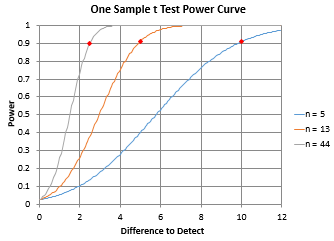# One/Two Sample Hypothesis TestingNeed to find out something about one or two samples hypothesis testing?

• What interval contains the true mean of my process?
• Are the averages of these two processes the same?
• Do these two test methods give the same result?
• What sample size do I need to detect a shift of one standard deviation?

## One/Two Sample Hypothesis Testing and SPC for Excel

Our SPC software, SPC for Excel, contains the following tests for one and two samples:

• One sample z and t tests for a mean
• One sample variance test
• z and t tests for the difference in two means
• t test for paired sample comparison
• One proportion test
• Two proportion test

The software also contains power and sample curves to help determine the sample size needed for a certain power. The output contains the major calculations and gives the conclusion, either the null hypothesis is rejected or not rejected based on the value of alpha.